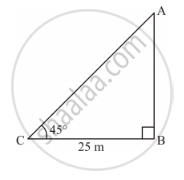Share

# A Tower Stands Vertically on the Ground. from a Point on the Ground Which is 25 M Away from the Foot of the Tower, the Angle of Elevation of the Top of the Tower is Found to Be 45°. - Mathematics

Course

#### Question

A tower stands vertically on the ground. From a point on the ground which is 25 m away from the foot of the tower, the angle of elevation of the top of the tower is found to be 45°. Then the height (in meters) of the tower is

##### Options
• A. 25sqrt2

• B. 25sqrt3

• C. 25

• D. 12.5

#### SolutionLet AB be the tower and C be the point on the ground 25 m away from the foot of the

tower such that ∠ACB = 45°.

In right ΔABC:

tan 45 ^@=(AB)/(BC)

rArr l=(AB)/25m

⇒ AB = 25 m

Thus, the height of the tower is 25 m.